# Logic blocks

Both Audia and Nexia have a large number of blocks that can be controlled or can report their state with logic signals. Those blocks can be audio blocks with logic control, control blocks and logic functions. The table below details all available blocks that use logic in Audia/Nexia and their functionality.

This article describes logic signals as being 1 or 0.  Note that a logic 1 is equivalent to a logic "on" or logic "high" state, and a logic 0 is equivalent to a logic "off" or logic "low" state.

Device

Logic I/O

OperationMute Control

1 logic input per audio channel, up to 16 channels per block

(56 ganged)

Logic input 1 causes audio channel to mute.

Logic input 0 causes audio channel to un-mute.Level Inc/Dec

1 logic input for level up (+) on each audio channel.

1 logic input for level down (-) on each audio channel.

Up to four audio channels per block (56 ganged)

Logic 1 input to (+) causes level to ramp up.

Logic 1 input to (-) causes level to ramp down.

Optional: Disable ramping

Transition from logic 0 to 1 on (+) causes audio to increment up.

Transition from logic 0 to 1 on (-) causes audio to increment down.Remote Preset

1 logic input per preset, up to 10 presets per block

Transition from logic 0 to logic 1 triggers preset to recall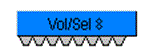Select 8

Volume/Select 8

8 logic inputs

Momentary button action

Button out = Logic 0

Button in= Logic 1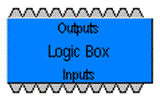Logic Box

20 logic ports, freely assignable as logic inputs or outputs

Open on logic input = Logic 1

Closure on logic input= Logic 0

Logic 1 on logic output = open

Logic 0 on logic input =closureVoltage Control Box

4 logic ports, freely assignable as logic inputs or outputs

Open on logic input = Logic 1

Closure on logic input= Logic 0

Logic 1 on logic output = open

Logic 0 on logic input =closure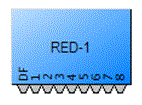RED-1

Up to 32 logic inputs

Logic 1 on DF = Device Found

Logic outputs normally 0. Transition to logic 1 for 250ms when button is pressedAuto Mixer

1 logic output per input channel, up to 32 channels per block

Logic output normally Logic 0

Switches to Logic 1 as long as corresponding input channel is gated on.

Optional: Behavior of logic output may be inverted in control dialog.Room Combiner

1 logic input per moveable partition, up to 32 rooms (58 partitions) per block

0 on logic input causes partition to remain open.

1 on logic input causes partition to remain closedPaging Zone Router

(N/A in Nexia)

1 Logic output per zone, up to 28 zones

Logic output normally Logic 0

Switches to Logic 1 when corresponding zone is being pagedSource Selection

1 Logic input per channel, up to 16 channels

1 Logic output per channel, up to 16 channels

Transition from logic 0 to 1 on logic inputs selects the corresponding audio source

Logic outputs normally logic 0. Logic 1 on logic output indicates corresponding audio source is selectedDucker

1 logic input and 1 logic output

1 on logic input causes program audio to duck and logic output to be 1.  0 causes program to un-duck.

Audio signal exceeding threshold on sense input causes logic output to be 1.  Audio signal below threshold causes logic output to be 0.

Optional: Behavior of logic control may be inverted in control dialog.1 logic input and 1 logic output

1 on logic input picks up the line or “takes off hook”. 0 causes the line to hang up or “Put back on hook”.

1 on logic output indicates line is ringing. 0 indicates line is not ringing.TI Dialer

19 logic inputs and 1 logic output

Transition from logic 0 to logic 1 on input 1 to 16 causes the associated number to be dialed.

Transition from logic 0 to logic 1 on Rdl input causes the last number to be redialed.

Transition from logic 0 to logic 1 on HF input causes a hook flash.

1 on DM input selects pulse dial mode. 0 on DM input selects tone dial mode.

1 on logic output indicates dialing in progress. 0 indicates no dialing in progress.TI DTMF Decode

17 logic outputs

Logic outputs 1 thru D pulse high (0 -> 1 -> 0) for about 250msec when the corresponding DTMF tone is detected.

Logic output Any pulses high (0 -> 1 -> 0) for about 250msec when any DTMF tone is detected.TI Control/Status

8 logic outputs

Logic 1 on HS = Off hook

Logic 0 on HS = On hook

Logic 1 on DTD = Dial tone detected

Logic 0 on DTD = No dial tone

Logic 1 on BTD = Busy tone detected

Logic 0 on BTD = Busy tone not detected

Logic 1 on RTD = Ring tone detected

Logic 0 on RTD = No ring tone detected

Logic 1 on LR = Line ready

Logic 0 on LR = Line not ready

Logic 1 on LIU = Line in use

Logic 0 on LIU = Line not in use

Logic 1 on LI = Line intrusion

Logic 0 on LI = No line intrusion

Logic 1 on LF = Line fault detected

Logic 0 on LF = No line faults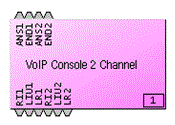VoIP-2 Console

(N/A in Nexia)

4 logic inputs and 6 logic outputs

Transition from 0 to 1 on ANS1 (ANS2) answers the call on line 1 (2)

Transition from 0 to 1 on END1 (END2) ends the call on line 1 (2)

Logic 1 on RI1 (RI2) = Line 1 (2) is ringing

Logic 0 on RI1 (RI2) = Line 1 (2) not ringing

Logic 1 on LIU1 (LIU2) = Line 1 (2) in use

Logic 0 on LIU1 (LIU2) = Line 1 (2) not in use

Logic 1 on LR1 (LR2) = Line 1 (2) in line ready mode

Logic 0 on LR1 (LR2) = Line 1 (2) in not ready modeNPS-1

(N/A in Nexia)

3 Logic outputs

Optional: 1 output per button

Logic 1 on CN = CobraNet connectivity is ok

Logic 0 on CN = No CobraNet connection or fault

Logic 1 on MG = Microphone is operational

Logic 0 on MG = Microphone fault (open or short)

Logic 1 on Any indicates a button is pressed (paging in process)

Optional: If logic outputs per button is selected, a logic 1 on any of the individual button logic outputs indicates button is pressedPA-2

(N/A in Nexia)

1 Logic output per amplifier channel (1 per pair in Bridge mode)

Logic output normally Logic 0

Switches to Logic 1 when corresponding amplifier channel faults (load and/or temperature fault)Fusion w/grouped logic

(Requires Fusion Device)

(N/A in Nexia)

1 Logic output per block plus 2 logic outputs per channel

All logic outputs normally logic 0

Logic 1 on FSR = Fan Stuck Rotor

Logic 1 on ALx = Alarm (Heat Sink OR Short Circuit OR Channel Failure)

Logic 1 on WNx = Warning (Heat Sink OR Excessive Clipping OR Low Impedance OR High Impedance)Fusion w/ungrouped logic

(Requires Fusion Device)

(N/A in Nexia)

1 Logic output per block plus 6 logic outputs per channel

All logic outputs normally logic 0

Logic 1 on FSR = Fan Stuck Rotor (Fan Failure)

Logic 1 on HSx = Heat Sink warning/alarm (Heat sink has reached 92% of cooling capacity)

Logic 1 on SCx = Short Circuit Alarm (Shorted load)

Logic 1 on CFx = Channel Failure Alarm (hardware failure)

Logic 1 on ECx = Excessive Clipping Warning (Clipping has exceeded the Clipping Count Threshold)

Logic 1 on LZx = Low Impedance Warning (Detected impedance is lower than allowed by the tolerance parameters)

Logic 1 on HZx = High Impedance Warning (Detected impedance is higher than allowed by the tolerance parameters)Fusion w/device failover and grouped logic

(Requires Fusion Device)

(N/A in Nexia)

3 Logic outputs per block plus 2 logic outputs per channel

All logic outputs normally logic 0 except PDG and SDG (see below)

Logic 1 on FSR = Fan Stuck Rotor

Logic 1 on PDG = Primary Device Good

Logic 0 on PDG = Primary Device Not Good (an Alarm is on in the primary device)

Logic 1 on SDG = Secondary Device Good

Logic 0 on SDG = Secondary Device Not Good (an Alarm is on in the secondary device)

Logic 1 on ALx = Alarm (Heat Sink OR Short Circuit OR Channel Failure)

Logic 1 on WNx = Warning (Heat Sink OR Excessive Clipping OR Low Impedance OR High Impedance)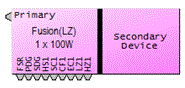Fusion w/device failover and ungrouped logic

(Requires Fusion Device)

(N/A in Nexia)

3 Logic outputs per block plus 6 logic outputs per channel

All logic outputs normally logic 0 except PDG and SDG (see below)

Logic 1 on FSR = Fan Stuck Rotor

Logic 1 on PDG = Primary Device Good

Logic 0 on PDG = Primary Device Not Good (an Alarm is on in the primary device)

Logic 1 on SDG = Secondary Device Good

Logic 0 on SDG = Secondary Device Not Good (an Alarm is on in the secondary device)

Logic 1 on HSx = Heat Sink warning/alarm (Heat sink has reached 92% of cooling capacity)

Logic 1 on SCx = Short Circuit Alarm (Shorted load)

Logic 1 on CFx = Channel Failure Alarm (hardware failure)

Logic 1 on ECx = Excessive Clipping Warning (Clipping has exceeded the Clipping Count Threshold)

Logic 1 on LZx = Low Impedance Warning (Detected impedance is lower than allowed by the tolerance parameters)

Logic 1 on HZx = High Impedance Warning (Detected impedance is higher than allowed by the tolerance parameters)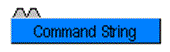Command String

1 logic input per command string, up to 8 per block

Transition from logic 0 to 1 triggers command string to output serial port.Event Scheduler

(N/A in Nexia)

1 logic input and 1 logic output per event, up to 8 per block

Logic output normally 0 and changes to 1 for 250 milliseconds at scheduled event time.

1 on logic input causes event scheduler to be disabled.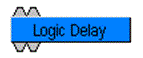Logic Delay

1 logic input and 1 logic output per delay, up to 8 per block

1 on logic input must be present for specified ON time before logic output changes to 1.

0 on logic input must be preset for specified OFF time before logic output changes to 0.OR Gate

2-8 logic inputs and 1 logic output

1 at either or both logic inputs causes a 1 at logic output.  Otherwise, logic output is 0.

Truth table:

 Input 1 Input 2 Output 0 0 0 0 1 1 1 0 1 1 1 1AND Gate

2-8 logic inputs and 1 logic output

1 at both inputs causes a 1 at logic output.  Otherwise, logic output is 0.

Truth table:

 Input 1 Input 2 Output 0 0 0 0 1 0 1 0 0 1 1 1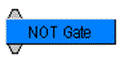NOT Gate

1 logic input and 1 logic output per gate, up to 8 per block

1 at logic input causes a 0 at logic output.

0 at logic input causes a 1 at logic output.

Truth table:

 Input Output 1 0 0 1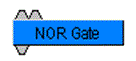NOR Gate

2-8 logic inputs and 1 logic output

0 at both logic inputs causes a 1 at logic output.  Otherwise, logic output is 0.  (Logically the same as an OR gate followed by a NOT gate.)

Truth table:

 Input 1 Input 2 Output 0 0 1 0 1 0 1 0 0 1 1 0NAND Gate

2-8 logic inputs and 1 logic output

1 at both logic inputs causes a 0 at logic output.  Otherwise, logic output is 1.

(Logically the same as an AND gate followed by a NOT gate.)

Truth table:

 Input 1 Input 2 Output 0 0 1 0 1 1 1 0 1 1 1 0XOR Gate

2-8 logic inputs and 1 logic output

1 at either but not both logic inputs causes a 1 at logic output.  Otherwise, logic output is 0.

Truth table:

 Input 1 Input 2 Output 0 0 0 0 1 1 1 0 1 1 1 0Flip Flop Gate

1 logic input and 1 logic output per gate, up to 8 per block

0 to 1 transition at logic input causes logic output to toggle from its present state to the opposite state.Logic Meter

1 logic input per meter, up to 16 per block

Logic 1 on input node turns corresponding indicator on

Logic 0 on input node turns corresponding indicator off